Engineering and Applied Sciences
Volume 1, Issue 2, August 2016, Pages: 20-25

Replica Exchange Using q-Gaussian Swarm Quantum Particle Intelligence Method

Hiqmet Kamberaj

Faculty of Engineering, Department of Computer Engineering, International Balkan University, Skopje, R. of Macedonia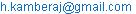Hiqmet Kamberaj. Replica Exchange Using q-Gaussian Swarm Quantum Particle Intelligence Method. Engineering and Applied Sciences. Vol. 1, No. 2, 2016, pp. 20-25. doi: 10.11648/j.eas.20160102.12

Received: June 15, 2015; Accepted: July 1, 2015; Published: July 16, 2016

Abstract: We present a newly developed Replica Exchange algorithm usingq -Gaussian Swarm Quantum Particle Optimization (REX@q-GSQPO) method for solving the problem of finding the global optimum. The basis of the algorithm is to run multiple copies of independent swarms at different values of q parameter. Based on an energy criterion, chosen to satisfy the detailed balance, we are swapping the particle coordinates of neighboring swarms at regular iteration intervals. The swarm replicas with high q values are characterized by high diversity of particles allowing escaping local minima faster, while the low q replicas, characterized by low diversity of particles, are used to sample more efficiently the local basins. We compared the new algorithm with the standard Gaussian Swarm Quantum Particle Optimization (GSQPO) and q-Gaussian Swarm Quantum Particle Optimization (q-GSQPO) algorithms, and found that the new algorithm is more robust in terms of the number of fitness function calls, and more efficient in terms of ability to convergence faster to the global minimum. In additional, we also provide a method for optimally allocating the swarm replicas among different q values. Our algorithm is tested for three benchmark functions, which are known to be multimodal problems, at different dimensionalities.

Keywords: Swarm Quantum Particle, q-Gaussian Distribution, Global Optimization, Replica Exchange

Contents

1. Introduction

The problem of finding the global optimum in a multimodal and multidimensional space can be extremely difficult since the number of stable optima increases as the search space increases, for instance, the search for the global minimum energy in a surface energy landscape of the atomic structures. [1,2] Swarm Particle Optimization (SPO) is a population-based optimization technique, similar to evolutionary algorithms.  Kennedy & Eberhart introduced the method to solve the problem of finding the global optimum of a q dimensional function.  The SPO method is based on the swarm intelligence algorithms, which concern with the design of intelligent multi-agent systems based on the collective behavior of insects (ants, termites, bees, and wasps) or other animal societies (flocks of birds and schools of fish). 

In SPO method, the swarm particles, representing possible solutions, search the phase space, defined by their velocities and coordinates, which are updated based on the particle’s own experience and experience of the particle’s neighbors or the experience of the whole swarm. The method has already been used to solve many optimization problems,  with some interests also in other fields, such as statistical mechanics. 

Since the standard SPO algorithm has a low convergence rate, [7,8] several improvements and variants of the SPO algorithm have been proposed. [9,10,11,12,13] The new variant of the SPO method, the so-called Swarm Quantum Particle Optimization (SQPO), has been considered as an improvement versus the classical SPO method, since there is a nonzero probability to escape the local minima even for very high barriers.  Efforts have been made to improve the SQPO method. [15,16,17,18,19,20,21,22] These improvements focus primarily on parameter selection criteria, [18,22] and maintaining diversity of the swarm. [19,20,21] A detailed review of all these methods is described in Ref.  Use of different forms of attractive potential-energy surfaces for SQPO algorithm is also considered for improvement of the algorithm.  Different potentials yield different probability distributions, which describe the probability of finding the swarm quantum-like particle at a certain position in the phase space. 

In our previous study , we showed that the use of q-Gaussian probability distribution of swarm particles (q-GSQPO algorithm) improved significantly the efficiency of searching for the global minimum when compared with Gaussian probability distribution of swarm particles (GSQPO algorithm). The q-Gaussian distribution is characterized by long tails, which allow reaching long distant regions in phase space by increasing the diversity of the swarm particles.  The application of the probability distributions with heavy tails is found to be useful in allowing the system escaping from local optima in multimodal problems also in other areas [25,26,27,28,29], since the nonzero occupations taken from long tails of the distribution implies jumps of scale-free sizes. The q - Gaussian distribution is also known as Tsallis distribution  in statistical mechanics, and it is known to produce a smoothed potential energy surface. 

The problem facing GSQPO algorithm is the premature convergence to a local minima due to low diversity of the swarm particles.  This problem of GSQPO algorithm can efficiently be solved by using the q-GSQPO algorithm , which increases the diversity between the swarm particles, and hence allowing the swarm to explore long distant regions of the searching space.

In this paper we will explore the use of the popular replica exchange method [33,34], which is known for overcoming the problem of the sampling convergence in dynamical systems. In this method several copies of the system (so-called replicas) are run independently at different values of some internal parameter of the system (e.g., temperature [34,35,36], strength of interaction , and q parameter when combined with Tsallis statistics [27,38]). At regular time intervals the coordinates of the replicas are swapped based on an energy criterion that satisfies the detailed balance. The higher parameter value replicas are used to enhance the barrier crossings and the low parameter value replicas are used to sample the local basins.

Similarly, in this study, we will create M copies of swarm particles (replicas) and run them independently at different values of q, where the lowest level replica is running at q=1. Then, in analogy with standard replica exchange methods, at regular iteration intervals, we swap the particles of swarm replicas between two neighboring values of q based on an energy criterion, which is explained in details in the next section. To increase the efficiency of the algorithm, we also discuss the optimization of the round-trip time of the swarm replicas from the lowest to the highest value of q and vice-versa. To test our algorithm, we studied three benchmark functions, namely the Ackley , Griewank  and Rastrigin  functions at different dimensions, d =5, 10, 20 and 50.

2. Materials and Methods

2.1. Theoretical Basics of Generalized q-GSQPO Algorithm

The swarm quantum particle optimization algorithm determines the probability of finding the swarm particle at the position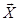at any time t.  Details of the derivation of GSQPO and q-GSQPO algorithms can be found in our previous work.  Here, we re-write the equations, proposed to describe the q-GSQPO algorithm in a generalized form as :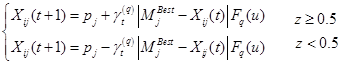(1)

where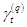has to be less than 1.7 in order to guarantee the convergence of the particles,  and it is chosen here to have a simplified sinusoidal expression as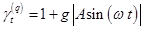(2)

with g being a scaling constant fixed in this study to 0.5,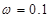, and A was varying from 0.01 to 1.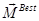, also called Mainstream Thought or Mean Best of the population , defines the mean of the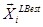best positions of all particles (i=1, 2, …, N) in each dimension and it is given by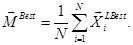(3)

Here, Fq(u) is a random number following q- Gaussian distribution if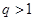and Gaussian distribution if q=1.

2.2. Replica Exchange Method

In the Replica Exchange q-GSQPO (REX@q-GSQPO) algorithm, we replicate M copies of the swarm particles among different values of q: q1, q2,…, qM. A geometrical distribution in the interval [1, qmax) was used to select the values of q, where qmax is a maximum value of q, which is chosen here to be between 2 and 3. In order to control the acceptance probability of swapping swarm particles between two neighboring q, we introduce a "temperature" parameter at each level: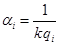(4)

where i=1,2,…,M. k is considered an adjustable parameter, which will be optimized to minimize the time of a round trip from the lowest to the highest value of q and vice-versa. At regular iteration steps we swap the configurations of swarm particles between two randomly chosen neighboring q, let say i and j, such that the detailed balance is satisfied: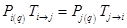(5)

where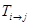and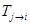are the transition probabilities from the swarm replica i to j and j to i, respectively. We will assume that the probability of finding the swarm i at a value q is proportional to the Boltzmann factor as: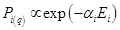(6)

where Ei is the minimum of the best local scoring value of the swarm particles in replica i at a given iteration step. Choosing Ei as the minimum of the best local scoring value is optional. Other choices may also be allowed, for instance, the mean best local scoring value or the best global scoring value of the replica. It is important that the choice should yield a high value of the diversity of swarm particles on each of replica in order to allow the swarm particles exploring more efficiently the searching space. This is in particular important for low q replicas, which are used to explore more efficiently the local basins.

Then, the probability of accepting an attempting swap between swarm replicas i and j is given by: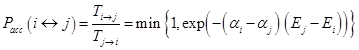(7)

The algorithm stops for searching the phase space when at least one of the swarm replicas converges to the optimal solution. In general, the global minimum of the problem is not known; therefore the searching does not have to stop when one of the replicas converges. For instance, one can restart the replica that has converged randomly from a new position. However, we found, for the problems under investigation, stopping the phase space search after the observation of the first replica converging to some optimal solution is one efficient way for determining criteria for stopping the algorithm.

3. Results and Discussions

We considered the motion in three d-dimensional potential functions determined mathematically as in Table 1.

Table 1. The three benchmark functions which are used in this study.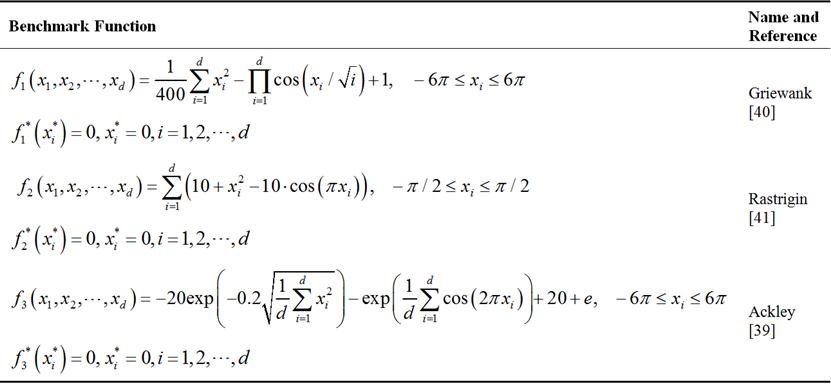In order to estimate the efficiency of the algorithm, we calculated the frequencies of visiting each q from each swarm replica and compared the observed frequencies for each swarm with expected frequencies, which depend only on the number of replicas. We used the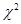test with a confidence level of 95 % to investigate the goodness of the comparison.  In this study we chose a geometrical distribution of q among 5 or 6 replicas. The replicated exchange simulations were stopped when the first global best score of the swarms was less than some minimum value, chosen here to be 10-5. As an estimation of the computational robustness of the method we used the number of iterations needed until the convergence was reached.

In addition, we also measured the highest and the lowest values of the diversity, which is given by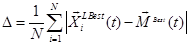(8)

Value of k will influence on the average allocation of the swarm replicas over the q space ladder, since the overlapping of probability distributions between two neighboring q values, and hence the acceptance probability of swapping the swarm replicas, depend on k. Due to the barriers that exist in the q space, the swarms at the low q values may not be able to visit the upper values and vice-versa. In order to adjust the value of k we did several test runs, and compared the computed frequencies of observing the swarms at a value of q with the expected frequencies assuming equal probability of finding a swarm at each value of q.

In Figure 1 we show the ratio of the computedwith critical value of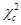at confidence level of 95 % for the three benchmark functions at different values of parameter k. The dimension the searching space was fixed to d=10. For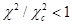, the probability distribution of swarm replicas in the q space is considered to be uniform, at a confidence level of 95 %, otherwise there is not enough evidence to say that this distribution is uniform. We found that for values of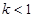, depending also on the benchmark function, there is enough evidence to say at the confidence level of 95 %, that the distribution of the swarm replicas in the q space is uniform (see Figure 1).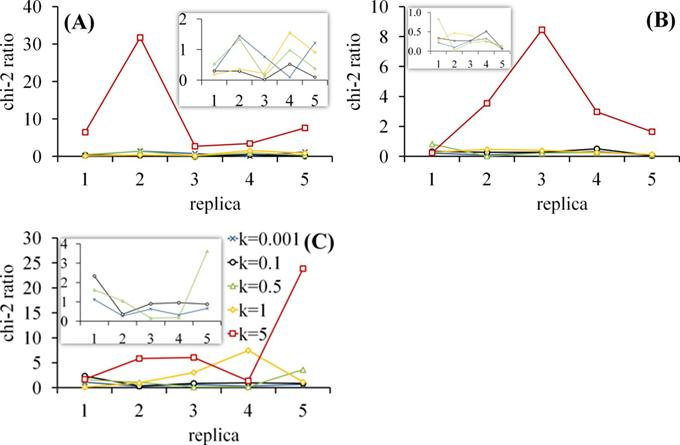Figure 1. The ratio between the calculated and critical value of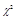at the confidence level of 95 % for different values of the parameter k. (A) For Ackley function; (B) Griewank function and (C) Rastrigin function. Dimensionality of the space was fixed to d=10, and q was chosen from a geometrical distribution in the range between one and two.

3.2. Robustness

In order to examine the robustness of the REX@q-GSQPO algorithm in comparison with other algorithms such as GSQPO and q-GSQPO in terms of function calls, we studied the number of iterations needed for each algorithm until the convergence was reached for a fixed value of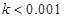. For the REX@q-GSQPO algorithm we replicated five swarms among five values of q in the range from 1 to 2 chosen from a geometrical distribution. The swapping of the swarm particle configurations between two neighboring values of q was performed at each iteration step. For the q-GSQPO algorithm we chose these values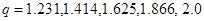. The results of the number of iterations for the GSQPO (q=1) and q-GSQPO algorithms were averaged over different values of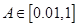.

Our results are presented graphically in Figure 2 for the three benchmark functions versus the search space dimension d (Ackley (A), Griewank (B) and Rastrigin (C)): in gray the average values for GSQPO and q-GSQPO algorithms, and in black for REX@q-GSQPO algorithm. Our data indicate that REX@q-GSQPO algorithm is more robust than GSQPO and q-GSQPO algorithms since the number of iterations needed for reaching the convergence is much smaller compare to GSQPO and q-GSQPO algorithms, in particular for high dimensionality which is of interest for many applications.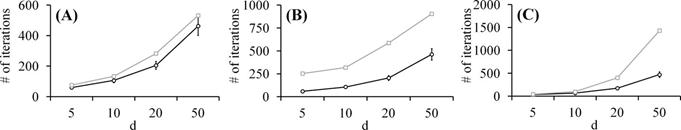Figure 2. The number of iterations as a function of the dimensionality of the problem d=5, 10, 20, 50 for REX@q-GSQPO (black line) and combined GSQPO and q-GSQPO (gray line) algorithms. (A) Ackley function. (B) Griewank function. (C) Rastrigin function.

3.3. Convergence

To examine the efficiency of the method on finding the global minimum, we investigated the average best score and compared the three algorithms presented here. We used the same setup as described above. In Figure 3 we are plotting the average best score for the REX@q-GSQPO algorithm (in black) and an average value for the GSQPO (q=1) and q-GSQPO (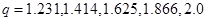) algorithms (in gray). Results are presented for the three benchmark functions and different dimensions of the searching space d. It can be seen that REX@q-GSQPO algorithm provides much smaller best scoring values compare to standard GSQPO and q-GSQPO algorithms. In addition, for the REX@q-GSQPO algorithm, in contrast to GSQPO and q-GSQPO algorithms, the convergence to the global minimum was achieved for all benchmark functions and for all dimensions considered here (see Figure 3).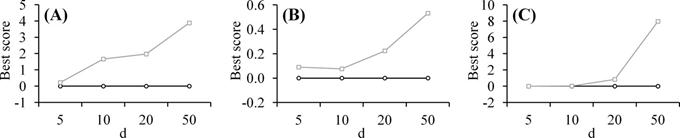Figure 3. The average best score value as a function of the dimensionality of the problem d=5, 10, 20, 50 for REX@q-GSQPO (black line) and combined GSQPO and q-GSQPO (gray line) algorithms. (A) Ackley function. (B) Griewank function. (C) Rastrigin function.

4. Conclusions

In this paper we presented a new algorithm for determining the global minimum of a multimodal problem using the replica exchange approach of the swarm quantum particles over the q searching space. The algorithm was compared with standard Gaussian swarm quantum particles and q-Gaussian swarm quantum particles algorithms in terms of the efficiency of convergence to the global minimum and computational robustness.

It was found the new algorithm, REX@q-GSQPO, outperforms the standard algorithms GSQPO and q-GSQPO in both, the efficiency of the convergence and the computational robustness. In addition, we also examine and showed how to optimize the probability distribution among q values in order to minimize the round-trip of the swarm replicas between the two extreme q values. This was an important step to ensure the ergodicity in the q space.

In the REX@q-GSQPO algorithm, the high q values swarm replicas, which are characterized by high diversity between the particles, can be used to explore distant regions in the sampling space, while the low q values, which are characterized by low diversity between the particles, can be used to sample efficiently the local basins.

We envision that the approach proposed in this study can be applied to other examples, as well, of swarm formation during collective migration including collective behaviour of biomolecular dynamics  by improving searching mechanism as proposed very recently  using the principle component analysis.

Acknowledgements

The author would like to thank the International Balkan University for the support.

References

1. O. Becker and M. Karplus, J. Chem. Phys. 106 (1997) 1495-1517.
2. D. Wales, Energy Landscapes with applications to clusters, biomolecules and glasses, Cambridge University Press, Cambridge, UK, 2003.
3. H. Schmeck and J. Branke, Designing evolutionary algorithms for dynamic optimization problems. Theory and application of evoluacionary computation: recent trends, S. Tsutsui and A. Ghosh, Eds., (2002) 239-262.
4. J. Kennedy, R. Eberhart and Y. Shi, Swarm Intelligence, Morgan Kaufmann, Los Altos, CA, 2001.
5. K. Parsopoulos and M. Vrahatis, Natural Computing, (2002) 235-306.
6. A. Walczak, W. Bialek, A. Cavagna, I. Giardina, T. Mora, E. Silvestri and M. Viale, Proc. Natl. Acad. Sci. USA. 109 (2012) 4786-4791.
7. T. Blackwell, R. Poli and J. Kennedy, Swarm Intell. 1 (2007) 33–57.
8. J. Kennedy, In Proceedings of the 2007 IEEE Swarm Intelligence Symposium, Honolulu, HI, 2007, pp. 162-169.
9. M. Clerc and J. Kennedy, Evolutionary Computation, IEEE Transactions on Evolutionary Computation 6 (2002) 58-73.
10. A. Banks, J. Vincent and C. Anyakoha, Natural Computing 6 (2007) 467-484.
11. A. Banks, J. Vincent and C. Anyakoha, Natural Computing 7 (2008) 109-124.
12. J. Sun, B. Feng and W. Xu, In the Proceedings of the 2004 IEEE congress on evolutionary computation, Piscataway, NJ, 2004, pp. 325-331.
13. J. Sun, B. Feng and W. Xu, In Proceedings of the 2004 IEEE conference on Cybernetics and Intelligent Systems, Singapore, 2004, pp. 111-116.
14. S. Mikki and A. Kishk, IEEE Trans. Antenn. Propag. 54 (2006) 2764–2775.
15. W. Xu, J. Liu and J. Sun, Quantum-behaved particle swarm optimization with adaptive mutation operator, Part I, Springer-Verlag, 2006.
16. W. Fang, J. Sun, Y. Ding, X. Wu and W. Xu, IETE Technical Review 27 (2010) 337-348.
17. J. Liu, J. Sun and W. Xu, Advances in Natural Computation 3612 (2005) 543-552.
18. J. Sun, W. Xu and B. Feng, In Proceedings of the 2005 IEEE International Conference on Systems, Man and Cybernetics, Waikoloa, HI, 2005, pp. 3049-3054.
19. J. Sun, W. Xu and W. Fang, In Proceedings of the 2006 Simulated Evolution and Learning Hefei, China, 2006, pp. 497-504.
20. J. Sun, W. Xu and W. Fang, In Proceedings of the 2006 Rough Sets and Current Trends in Computing, Kobe, Japan, 2006, pp. 736-745.
21. J. Sun, W. Xu and W. Fang, In Proceedings of the 2006 International Conference on Computational Science, Springer, Berlin, Heidelberg, 2006, pp. 847-854.
22. W. Xu, M. Xi and J. Sun, Appl. Math. Comput. 205 (2008) 751-759.
23. W. Xu, W. Fang, J. Sun, Y. Ding and X. Wu, IETE Technical Review 27 (2010) 336-348.
24. H. Kamberaj, Appl. Math. Comput. 229 (2014) 94-106.
25. J. Straub and I. Andricioaei, Brazilian J. Phys. 29 (1999) 179-186.
26. D. Stariolo and C. Tsallis, Annual Reviews of Computational Physics II, editied by D. Stauffer, Singapore, World Scientific, 1995.
27. H. Kamberaj and A. van der Vaart, J. Chem. Phys. 127 (2007) 234102-7.
28. C. Tsallis and D. Stariolo, Physica A 233 (1996) 395-406.
29. S. Umarov, C. Tsallis and S. Steinberg, Milan J. Math. 76 (2008) 307-328.
30. C. Tsallis, J. Stat. Phys. 52 (1988) 479-487.
31. I. Andricioaei and J. Straub, J. Chem. Phys. 107 (1997) 9117-9125.
32. J. Riget and J. Vesterstroem, A diversity-guided particle swarm optimizer - the ARPSO, Department of Computer Science, University of Aarhus, 2002.
33. K. Hukushima and K. Nemoto, J. Phys. Soc. (Jpn) 65 (1996) 1604-1607.
34. R. H. Swendsen and J.-S. Wang, Phys. Rev. Lett. 58 (1987) 86-88.
35. Y. Okamoto and Y. Sugita, Chem. Phys. Lett. 314 (1999) 141-151.
36. H. Kamberaj and A. van der Vaart, J. Chem. Phys. 130 (2009) 074906.
37. H. Fukunishi, O. Watanabe and S. Takada, J. Chem. Phys. 116 (2002) 9058-9068.
38. J. Kim and J. Straub, J. Chem. Phys. 130 (2009) 144114-11.
39. D. Ackley, A connectionist machine for genetic hillclimbing, Kluwer Academic Publishers, Boston, 1987.
40. A. Griewank, J. Optimiz. Theory App. 34 (1981) 11-39.
41. P. Angeline, Evolutionary Optimization Versus Particle Swarm Optimization: Philosophy and Performance Differences, Evolutionary Programming VII, Lecture Notes in Computer Science, Springer, Berlin, Heidelberg, New York, 1998.
42. L. Meester, F. Dekking, C. Kraaikamp and H. Lopuhaa, A Modern Introduction to Probability and Statistics, Springer-Verlag, London, 2005.
43. H. Kamberaj, Cent. Eur. J. Phys. 9 (2011) 96-109.
44. X. Zhao, W. Lin and Q. Zhang, Appl. Math. Comput. 229 (2014) 440-456.

 Contents 1. 2. 2.1. 2.2. 3. 3.1. 3.2. 3.3. 4.
Article ToolsAbstractPDF(1313K)